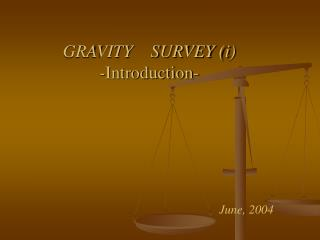DownloadDownload PresentationGRAVITY SURVEY (i) - Introduction-

GRAVITY SURVEY (i) - Introduction-

Télécharger la présentationGRAVITY SURVEY (i) - Introduction-

- - - - - - - - - - - - - - - - - - - - - - - - - - - E N D - - - - - - - - - - - - - - - - - - - - - - - - - - -
Presentation Transcript

1. GRAVITY SURVEY (i)-Introduction- June, 2004

2. Gravity Survey • Measurements of the gravitational field at a series of different locations over an area of interest. The objective in exploration work is to associate variations with differences in the distribution of densities and hence rock types. Occasionally the whole gravitational field is measured or derivatives of the gravitational field, but usually the difference between the gravity field at two points is measured (From: R. E. Sheriff; Encyclopedic Dictionary of Exploration Geophysics, published by the Society of Exploration Geophysics )

3. In gravity prospecting, geophysicists measure minute variations in the force of gravity from rocks up to a few miles beneath the earth's surface. • Different types of rocks have different densities, and the denser rocks have the greater gravitational attraction.

4. Theory of gravity Use two of Newton’s laws: Universal law of gravitation: Where G=6.67 ´ 10-11 N m2/kg2 (m3/kg.s2) Second law of motion: F = mg We can combine them to obtain the gravitational acceleration at the surface of the earth:

5. Gravity and potentials g is a vector field: where r1 is the unit vector pointing toward the center of the Earth Gravitational potential: U is a scalar field which makes it easier to work with g is a vector field: Definition:The gravitational potential, U, due to a point mass m, at a distance r from m, is the work done by the gravitational force in moving a unit mass from infinity to to a position r from m.

6. Relating g to U U is a scalar field which makes it easier to work with: • Potentials are additive • Gravity is a conservative force • And gravitational acceleration can be easily determined from the potential… It follows that: For smaller scale problems we usually deal with g, and sum the vertical component of g

7. Units for g SI unit for g: m/s2 1 cm/s2 = 1 Gal (for Galileo) = 0.01 m/s2 milliGal or mGal = 10-3 Gal – typical unit for field studies some text book uses the “gravity unit” (g.u.) 1 g.u. = 0.1 mGal Normal value of g at the surface of the Earth: gE = 9.8 m/s2 = 980 cm/s2 = 980 Gal = 980,000 mGal = 9800 g.u.

8. Gravity Meter A gravimeter is any instrument designed to measure spatial variations in gravitational acceleration

9. THANK YOU Question? Gravity 1/7